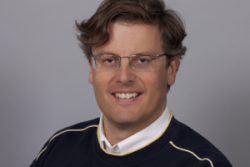# Research

## Calculus of Variations and Nonlinear PDE-Systems

Many problems in analysis, geometry, physics, engineering, and economics can be cast into the form of minimizing a functional I(u) among a class of admissible functions u. Important early examples of such functionals minimized in nature are: time of travel of a light ray (Fermat's principle in optics, 1662), action of a trajectory of a mechanical system (Hamilton's principle, 1834), and energy of the electrostatic field outside a charged body (Dirichlet's principle, Dirichlet, Kelvin, Gauss, 1840s). Minimizers of the latter problem solve Laplace's equation Δu=0, linking the calculus of variations to the theory of partial differential equations. Many fascinating modern day minimization problems can be viewed as far-reaching nonlinear extensions of Dirichlet's principle, in that minimizers solve nonlinear partial differential systems of equations.

In particular my interest is about existence and regularity of nonlinear PDE-Systems deriving from engineering applications and structural mechanics, as e.g., plasticity problems, extended continuum mechanics, shells and plates etc. Useful tools are newly derived coercive inequalities, like a generalized Korn‘s inequality or an inequality connecting the curl of a rotation to all partial derivatives. The precise amount of regularity of the (weak) solution is also a decisive information for subsequent FEM-implementations.

I have first applied successfully the fundamental notion of John Balls polyconvexity to materials undergoing large deformations with preferred directions (anisotropy). A specific application is e.g. biological tissue. Thus I have partly answered problem 2/18 of John Ball‘s „Some open problems in elasticity“: „Are there ways to verifying polyconvexity and quasiconvexity for a useful class of anisotropic stored energy functions?“ This topic is part of ongoing research, fully funded by the DFG.

I am also interested in 3D-to-2D reduction of nonlinear elasticity theory to membrane-, plate- and shell theories. A main mathematical tool here is Gamma convergence, introduced by De Giorgi and developed notably by Dal Maso, Braides and coworkers. It provides a powerful and rigorous mathematical framework to pass from a finer-scale (or higher-dimensional) variational principle to a coarser-scale (or lower-dimensional) effective variational principle.

Plasticity, either small strain or large strain, takes a prominent role in my investigations. I have developed a novel model for a geometrically exact description based on the multiplicative decomposition of the deformation gradient into elastic and plastic parts with viscoelastic effects for which well-posedness is shown by the above mentioned methods. In this field this is one of the view results establishing the existence of classical, smooth solutions. In small strain plasticity, I have extended the Cosserat model to incorporate plastic effects and more recently I have used variational inequalities to show existence for a weak reformulation of gradient plasticity.

The mathematics of extended continuum models is another field of research. Here, I treat e.g. Cosserat and micromorphic models which may arise through certain homogenization schemes from small scale to large scale, think e.g. of a continuum description of a metallic foams. I have set up a new finite strain Cosserat model and I have shown existence of minimizers. A major challenge here is given by the nonlinear structure of the group of rotations SO(3). Recently, I have also re-examined the small strain Cosserat model with very weak curvature conditions, thus eliminating some troubling physical inconsistency problems of the model.

## Numerical Methods

Attendant to formulating mathematically sound models I am interested in putting these models to work through Finite-Element simulations. While I am not coding myself I am treating FEM-convergence issues from a theoretical point of view e.g. in plasticity or in discontinuous Galerkin-Methods where I have shown optimal BV-convergence for linear elasticity. I am also interested in the numerical methods for shells, plates and membranes.

## Selected contributions

• A.Lew, P. Neff, D. Sulsky, and M. Ortiz. Optimal BV estimates for a discontinuous Galerkin method for linear elasticity. Applied Mathematics Research Express 3:73– 106, 2004. AMRX- Full Text (PDF), TUD-Preprint Nr. 2300, DOI
• P.Neff and I. Münch. Curl bounds Grad on SO(3). ESAIM: Control, Optimisation and Calculus of Variations, 14(1):148–159, 2008. DOI, TUD-Preprint Nr. 2455
• P.Neff and D. Knees. Regularity up to the boundary for nonlinear elliptic systems arising in time-incremental inﬁnitesimal elasto-plasticity. SIAM J. Math. Anal. 40(1):21-43, 2008. DOI , TUD-Preprint Nr. 2520

## My research interests can be summarized as follows:

Analysis:  Partial Differential Equations, Coercive Inequalities, Variational Methods, Elliptic Regularity, Applied Functionalanalysis, Evolution Equations, Polyconvexity, Convex Analysis

Numerics:  Finite-Element Methods, Discontinuous Galerkin, FETI-DP

Dimension Reduction:  Asymptotic Methods, Homogenisation, Gamma-Convergence, Theory of Shells, Plates and Membranes

Mathematical Aspects of Material Science, Continuum Mechanics:  Finite Strain Elasticity,  Finite Strain Plasticity, Gradientplasticity, Size-Effects, Microstructures

Extended Continuum Mechanics:  Cosserat, Micropolar and Micromorphic Models

## DFG-Research Project

Currently there is one third-party fully funded DFG-Research Project:
NE 902/2-1 and SCHR 570/6-1 (Neff/Schröder)
„Polykonvexe Energiefunktionen im Rahmen der Anisotropen Elastizität“,

1.Förderperiode 2006-2008  Zwischenbericht ,
2.Förderperiode 2008-2010

Application for third-party funding (October 2008) in the framework of
DFG-SPP 1420: Biomimetic Materials Research: Functionality by Hierarchical Structuring of Materials, together with M. Epple, A. Klawonn and A. Rösch
„Modelling, numerical simulation, optimization and experimental design of a hierarchically structured polymer.“

I am grateful for longstanding prolific working relations with

## Prof. Dr. rer. nat. habil. Patrizio Neff

Tel. +49(0)201/183-4243
patrizio.neff@uni-due.de

Room WSC-S-4.13## Contact

Universität Duisburg-Essen
Fakultät für Mathematik
Mathematik-Carrée
Thea-Leymann-Straße 9
45127 Essen

## Office hours

always if present